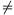Mathematical and Physical Journal
for High Schools
Issued by the MATFUND Foundation
 Already signed up? New to KöMaL?

#Problem A. 564. (May 2012)

A. 564. Let k be the incircle in the triangle ABC, which is tangent to the sides AB, BC, CA at the points C0, A0 and B0, respectively. The angle bisector starting at A meets k at A1 and A2, the angle bisector starting at B meets k at B1 and B2; AA1<AA2 and BB1<BB2. The circle k1k is tangent externally to the side CA at B0 and it is tangent to the line AB. The circle k2k is tangent externally to the side BC at A0, and it is tangent to the line AB. The circle k3 is tangent to k at A1, and it is tangent to k1 at point P. The circle k4 is tangent to k at B1, and it is tangent to k2 at point Q. Prove that the radical axis between the circles A1A2P and B1B2Q is the angle bisector starting at C.

(5 pont)

Deadline expired on June 11, 2012.

### Statistics:

 1 student sent a solution. 5 points: Mester Márton.

Problems in Mathematics of KöMaL, May 2012